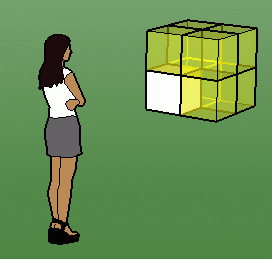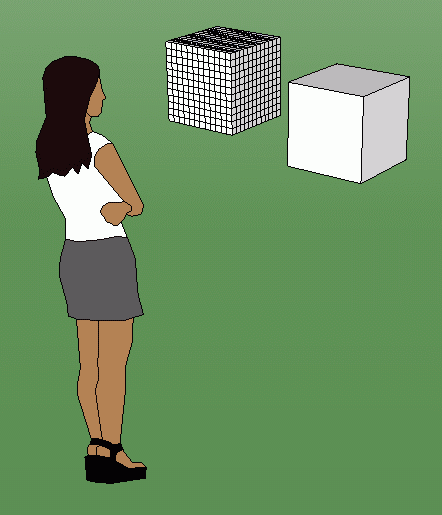SEARCH HOMEMath Central Quandaries & QueriesQuestion from carol, a parent: volume of cube = 216 cubic inches how do I calculate cubic feet  and calculate its surface area in square feet. a rectangular box has dimensions of 3 x 6 x 15 how do I calculate surface area in square yards and calculate volume in cubic inches. if I double the dimensions how does it affect the volumeHi Carol.

This set of problems is trying to teach you that when you change linear (distance) units, the corresponding area and volume units don't change by the same factor. The area changes by the square of the distance factor and the volume changes by the cube of the distance factor.So if I have a white box that is 1 foot by 1 foot by 1 foot and I double all the dimensions, is the surface area doubled? Is the volume doubled? No.

The surface area of a 1 foot by 1 foot by 1 foot cube is 6 sides each with the area 1 foot x 1 foot. So that is 6 x 1 x 1 = 6 square feet. The volume is 1 foot x  1 foot x  1 foot = 1 cubic foot.

Now let's double the dimensions (yellow):

The surface area is now 6 sides each with the area 2 feet x 2 feet. So that is 6 x 2 x 2 = 24 square feet. The volume is now 2 feet x 2 feet x 2 feet = 8 cubic feet.

Therefore, when the lengths went up by a factor of 2 (we went from 1 foot to 2 feet), the surface area went up by a factor of 4 and the volume went up by a factor of 8. You can see that 22 = 4 and 23 = 8, so we can say that when the distance is changed by a factor, the area is changed by the square of the factor and the volume is changed by the cube of the factor.Changing units:

When we change units (rather than the size), we are also just applying a factor. For example, if I have a box that is 1 foot by 1 foot by 1 foot, then it is 12 inches by 12 inches by 12 inches.

I haven't changed the box, so its surface area and volume are the same, but I can calculate them in inches: surface area is 6 x 12 x 12 =  864 square inches and volume is 12 x 12 x 12 = 1728 cubic inches.

Now let's look at a 1 foot by 1 foot by 1 foot cube in equation form:

 Volume: 1 cubic foot = 1 foot3 = 1 x (1 foot)3 = 1 x (12 inches)3 = 1 x 123 inches3 = 1728 inches3 = 1728 cubic inches. Surface area : 6 square feet = 6 feet2 = 6 x (1 foot)2 = 6 x (12 inches)2 = 6 x 122 inches2 = 864 inches2 = 864 square inches.

If you were going from a smaller unit to a larger unit (for example, from feet to yards), you would use a fraction as the factor: 1 foot = 1/3 yard. So 1 cubic foot = (1/3)3 yards3 = 1/27 cubic yards.

Hope this helps you,
Stephen La Rocque.Math Central is supported by the University of Regina and The Pacific Institute for the Mathematical Sciences.